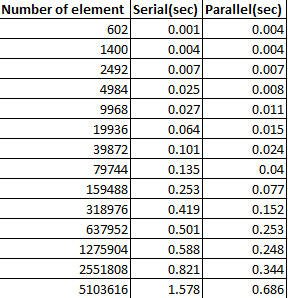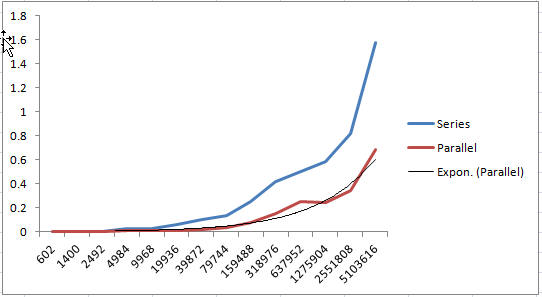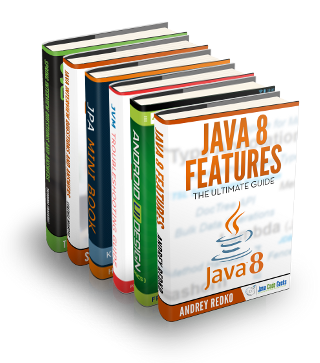Home » Java » Core Java » Arrays.sort versus Arrays.parallelSort# Arrays.sort versus Arrays.parallelSort

We all have used Arrays.sort to sort objects and primitive arrays. This API used merge sort OR Tim Sort underneath to sort the contents as shown below:

```public static void sort(Object[] a) {
if (LegacyMergeSort.userRequested)
legacyMergeSort(a);
else
ComparableTimSort.sort(a);
}```

This is all done sequentially, even though merge sort uses divide and conquer technique, its all done sequentially. Come Java 8, there is a new API introduced for sorting which is Arrays#parallelSort. This is does the sorting in parallel. Interesting right! Lets see how it does…

Arrays#parallelSort uses Fork/Join framework introduced in Java 7 to assign the sorting tasks to multiple threads available in the thread pool. This is called eating your own dog food. Fork/Join implements a work stealing algorithm where in a idle thread can steal tasks queued up in another thread.

## An overview of Arrays#parallelSort:

The method uses a threshold value and any array of size lesser than the threshold value is sorted using the Arrays#sort() API (i.e sequential sorting). And the threshold is calculated considering the parallelism of the machine, size of the array and is calculated as:

```private static final int getSplitThreshold(int n) {
int p = ForkJoinPool.getCommonPoolParallelism();
int t = (p > 1) ? (1 + n / (p << 3)) : n;
return t < MIN_ARRAY_SORT_GRAN ? MIN_ARRAY_SORT_GRAN : t;
}```

Once its decided whether to sort the array in parallel or in serial, its now to decide how to divide the array in to multiple parts and then assign each part to a Fork/Join task which will take care of sorting it and then another Fork/Join task which will take care of merging the sorted arrays. The implementation in JDK 8 uses this approach:
– Divide the array into 4 parts.
– Sort the first two parts and then merge them.
– Sort the next two parts and then merge them.
And the above steps are repeated recursively with each part until the size of the part to sort is not lesser than the threshold value calculated above.

## Some interesting results:

I tried to compare the time taken by the Arrays#sort and Arrays#parallelSort on a machine with 4 CPUs. The program which I used for this comparison is:

```public class ArraysParallelDemo {
public static void main(String[] args) throws FileNotFoundException {
List<Double> arraySource = new ArrayList<>();

getSystemResourceAsStream("java8demo/large_array_input"));
String[] strNums = line.split(",");
for ( String strN : strNums){
}
}

System.out.println(arraySource.size());

Double [] myArray = new Double;
myArray = arraySource.toArray(myArray);
long startTime = System.currentTimeMillis();
Arrays.sort(myArray);
long endTime = System.currentTimeMillis();
System.out.println("Time take in serial: "+
(endTime-startTime)/1000.0);

Double [] myArray2 = new Double;
myArray2 = arraySource.toArray(myArray);
startTime = System.currentTimeMillis();
Arrays.parallelSort(myArray2);
endTime = System.currentTimeMillis();
System.out.println("Time take in parallel: "+
(endTime-startTime)/1000.0);

}
}```

And the time taken by each of the APIs against arrays of double values of different sizes is shown below:There is a similar implementation for Lists as well and lot of the operations on Lists have a parallel equivalent.

Reference: Arrays.sort versus Arrays.parallelSort from our JCG partner Mohamed Sanaulla at the Experiences Unlimited blog.(0 rating, 0 votes)
You need to be a registered member to rate this.
1 Comment Views Tweet it!
Do you want to know how to develop your skillset to become a Java Rockstar?
Subscribe to our newsletter to start Rocking right now!
To get you started we give you our best selling eBooks for FREE!
1. JPA Mini Book
2. JVM Troubleshooting Guide
3. JUnit Tutorial for Unit Testing
4. Java Annotations Tutorial
5. Java Interview Questions
6. Spring Interview Questions
7. Android UI Design
and many more ....Subscribe
Notify ofThis site uses Akismet to reduce spam. Learn how your comment data is processed.

1 Comment
Inline Feedbacks